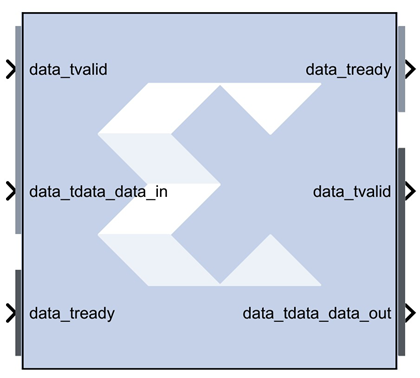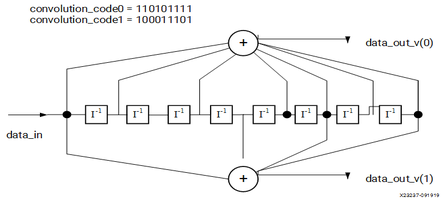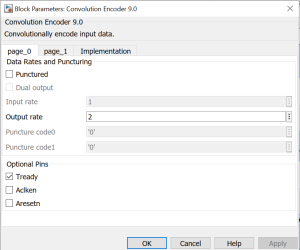# Convolution Encoder 9.0 - 2022.1 English

## Vitis Model Composer User Guide (UG1483)

Document ID
UG1483
Release Date
2022-05-26
Version
2022.1 English

The Xilinx® Convolution Encoder block implements an encoder for convolution codes. Ordinarily used in tandem with a Viterbi decoder, this block performs forward error correction (FEC) in digital communication systems. This block adheres to the AMBA® AXI4-Stream standard.Values are encoded using a linear feed forward shift register which computes modulo-two sums over a sliding window of input data, as shown in the figure below. The length of the shift register is specified by the constraint length. The convolution codes specify which bits in the data window contribute to the modulo-two sum. Resetting the block will set the shift register to zero. The encoder rate is the ratio of input to output bit length; thus, for example a rate 1/2 encoder outputs two bits for each input bit. Similarly, a rate 1/ 3 encoder outputs three bits for each input bit.

Figure 1. Linear Feed Forward Shift Register## Block Parameters

The following figure shows the Block Parameters dialog box.

Figure 2. Block Parameter Dialog Boxpage_0 tab
Parameters specific to the page_0 tab are as follows.
Data Rates and Puncturing
Punctured
Determines whether the block is punctured.
Dual Output
Specifies a dual-channel punctured block.
Input Rate
Punctured: Only the input rate can be modified. Its value can range from 2 to 12, resulting in a rate n/m encoder where n is the input rate and n<m<2n.
Output Rate
Not Punctured: Only the output rate can be modified. Its value can be integer values from 2 to 7, resulting in a rate 1/2 or rate 1/7 encoder, respectively
Puncture Code0 and Code1
The two puncture pattern codes are used to remove bits from the encoded data prior to output. The length of each puncture code must be equal to the puncture input rate, and the total number of bits set to 1 in the two codes must equal the puncture output rate (m) for the codes to be valid. A 0 in any position indicates that the output bit from the encoder is not transmitted. See the associated LogiCORE™ data sheet for an example.
Optional Pins
Adds a tready pin to the block. Indicates that the slave can accept a transfer in the current cycle.
Aclken
Adds a aclken pin to the block. This signal carries the clock enable and must be of type Bool.
Aresetn
Adds a aresetn pin to the block. This signal resets the block and must be of type `Bool`. The signal must be asserted for at least 2 clock cycles, however, it does not have to be asserted before the decoder can start decoding. If this pin is not selected, Model Composer ties this pin to inactive (high) on the core.
page_1 tab
Parameters specific to the page_1 tab are as follows.